# The Magic of the “Golden Ratio”

Walking around NYC, I was on a mission to connect mathematics to the real world.  This, of course, led me to go on a mathematical scavenger hunt in search of  the “Golden Ratio.” Hidden in plain sight, this often times naturally occurring ratio is seen everywhere from historic and modern architecture to nature itself.

What is this all-encompassing “Golden Ratio” you may ask?
It’s a proportion, related to a never-ending sequence of numbers called the Fibonacci sequence, and is considered to be the most pleasing ratio to the human eye.  The ratio itself is an irrational number equal to 1.618……..(etc.).

Why should you care?
When the same ratio is seen in the Parthenon, the Taj Mahal, the Mona Lisa and on the shores of a beach in a seashell, you know it must be something special!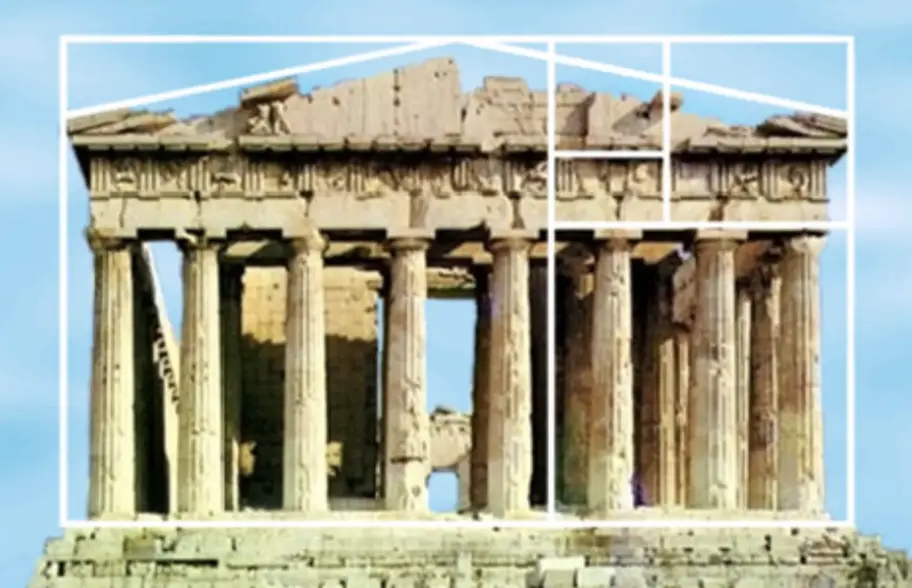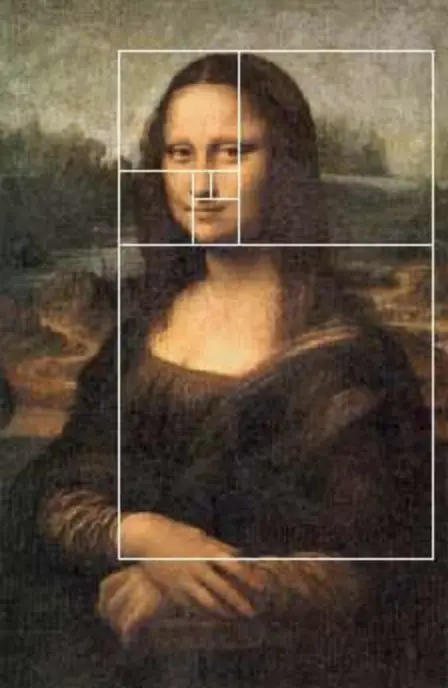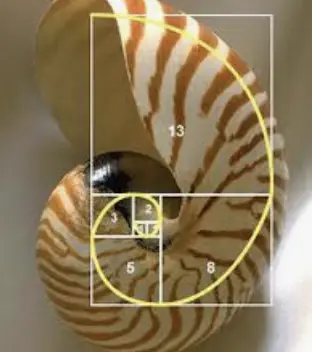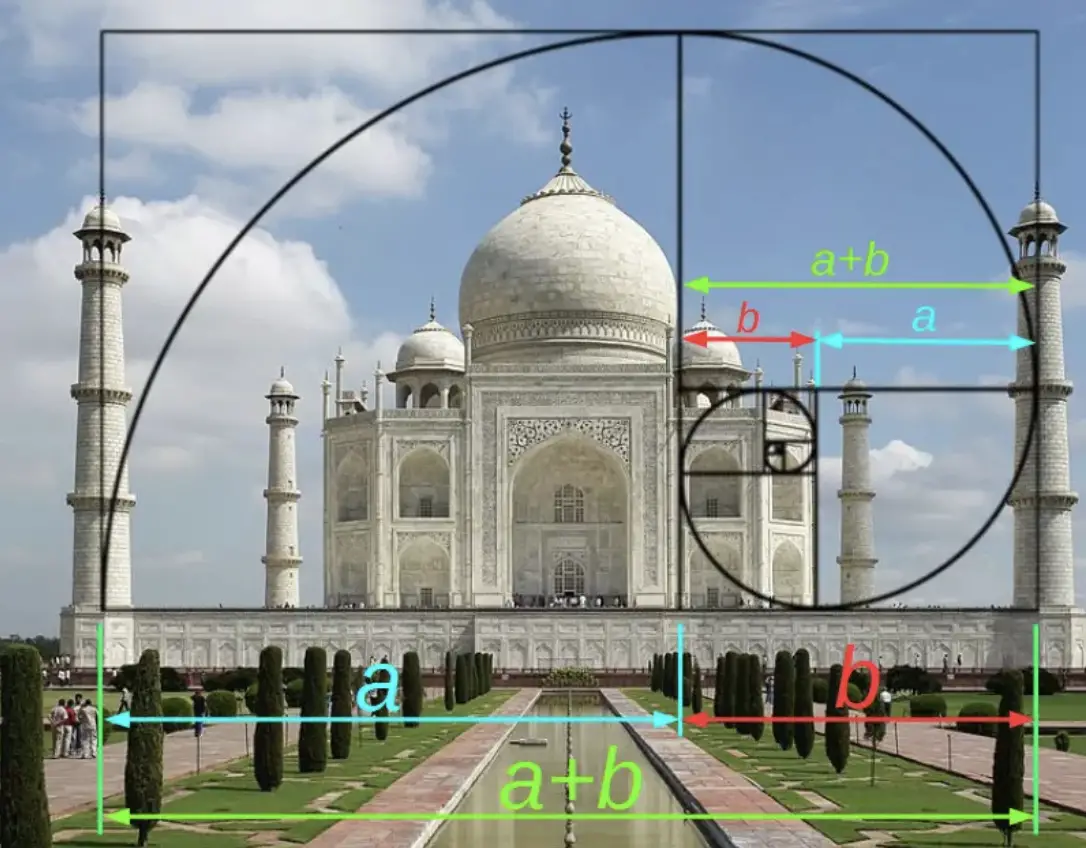Random as it may seem, this proportion stems from the following sequence of numbers, known as the Fibonacci sequence:

1, 1, 2, 3, 5, 8, 13, 21, …….

Do you notice what pattern these numbers form?
(Answer: Each previous two numbers are added together to find the next number.)

The Golden RectangleThe most common example of the “Golden Ratio” can be seen in the Golden rectangle.  The lengths of this rectangle are in the proportion from 1: 1.618 following the golden ratio. Behold the beauty of the Golden Rectangle:

How is the Fibonacci Sequence related to the Golden Ratio?                                               What if we drew a golden rectangle within our rectangle?

Then drew another golden rectangle within that golden rectangle?

And we kept doing this until we could no longer see what we were doing…….

The proportion between the width and height of these rectangles is 1.618 and can also be shown as the proportion between any two numbers in the Fibonacci sequence as the sequence approaches infinity. Notice that the area of each rectangle in the Fibonacci sequence is represented below in increasing size:

Where exactly can you find this Golden Ratio in real life? Found in NYC! The Golden ratio was seen here at the United Nations Secretariat building in the form of a golden rectangle(s).  Check it out!

Where have you seen this proportion of magical magnitude?  Look for it in your own city or town and let me know what you find! Happy Golden Ratio hunting! 🙂

If you’re interested in learning more about the golden ratio and are also a big Disney fan, I highly recommend you check out Donald Duck’s Math Magic!

Don’t forget to connect with MathSux on these great sites!

Posted on Categories Uncategorized

## 12 thoughts on “The Magic of the “Golden Ratio””

1.T Haag says:

Thanks for the Mona Lisa mini-lesson!

1.Math Sux says:

Glad you liked it! Thanks for stopping by!

2.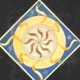Monty says:

My kids are fascinated with the golden rectangle

1.Math Sux says:

It is a very interesting mathematical phenomenon. Thanks for stopping by!